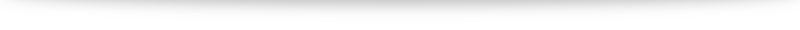# Q6b discussion

Continuing from your method (making sure to use a new letter), we have $$\frac{a^2-b^2-2abi}{a+bi} = 0 + ic$$ Simplifying gives us $a^2-b^2 - 2abi = -bc + aci$. It looks very unwieldy, but let's use our usual techniques (comparing real and imaginary parts). Our final aim is to find $w$ in terms of $a$ so let's find $b$ and $c$ in terms of $a$. Give it a try first, and you can check against my working below:
We have already calculated that $\displaystyle \frac{(w^*)^2}{w} = \frac{a^2-b^2-2abi}{a+bi}$. Notice there is a division in our working, so we can further simplify ("rationalize" using conjugates)! Give it a try.